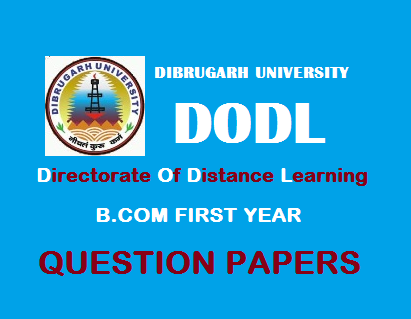# B.Com First Year Question Papers (Distance): Cost Accounting' 20161 TDC CA 7 (DE)
2016
(July)
COMMERCE
Paper: 101
(Cost Accounting)
Full Marks: 90
Time: 3 hours
The figures in the margin indicate full marks for the questions

1. (A) Write True or False: 1x4=4
1. Fixed cost per unit decreases with rise in output and increases with fall in output.
2. The method of costing used in an oil refinery is Job Costing.
3. High Wages need not necessarily mean high cost per unit.
4. In contract costing, most of the items of cost are direct.
(B) Choose the correct answer: 1x2=2

1. Administrative expenses are mostly fixed/variable.
2. High/low labour turnover rate denotes good human relations.
(C) Fill in the blanks: 1x4=4
1. ____ is the combination of direct material, direct labour and direct expenses.
2. Where actual loss in a process is less than the anticipated loss the difference between the two is considered to be ____.
3. Variable costs change ____ with changes in production.
4. Depreciation on showroom building is to be treated as ____ overheads.
1. State five differences between Cost Accounting and Financial Accounting.
2. Discuss the essentials of an ideal cost accounting system.
3. Explain the necessity of reconciliation between Cost and Financial Accounts.
4. What are the differences between Normal Loss and Abnormal loss in process costing?
5. Explain the salient features of ‘Rawan Plan’ and ‘Halsey Plan’.
3. (a) Describe the various methods of pricing material issues and point out their advantages and disadvantages. 7+8=15

Or
(b) The details of two Materials A and B used in a factory are as below: 3x5=15
1. Maximum usage – 600 units per week each
2. Minimum usage – 250 units per week each.
3. EOQ – A – 1,300 units, B – 2,500 units.
4. Lead Time A – 1 to 2 months, B – 2 to 3 months.
Calculate for each material –
1. Re-order Level.
2. Maximum Level.
3. Minimum Level.
4. Average Stock Level.
5. Danger Level [earliest possible time for supply A – 2 weeks, B – 3 weeks.]
4. (a) What is the meaning of ‘Labour Turnover’? State the causes of such labour turnover. How does it effect on efficiency of labour? 3+6+6=15
Or
(b) A worker on a day’s wage of Rs. 72 for, 8 hours is given a job to be completed. He is also given Re. 1 per hour actually worked as cost of living bonus. If he takes 6 hours to complete the job, what is his total earnings under Halsey Plan? What difference would it make if he is given under Rowan Plan? 6+7+2=15

5. Define overhead. What do you mean by absorption of overheads? Discuss the different methods of absorption of overheads. 4+3+8=15
6. (a) A product passes through three processes A, B, and C. The normal wastage of each processes is 3%, 5%, and 8% respectively. The wastage of each process is sold for Rs. 75, Rs. 238 and Rs. 728 respectively.
10,000 units were issued to process A in the beginning of the month of Re. 1 per unit. The other expenses were as follows: 15
 A(Rs.) B(Rs.) C(Rs.) Sundry materials Labour Direct Expenses 1,0008,000475 3,00013,0001,338 5005,300388

The actual was A – 9,500 units, B – 9,100 units and C – 8,100 units. There was no stock at beginning or end of the month. Prepare Process Accounts.
Or
(b) What is Operating Costing? What are its characteristics? In which industries is this method applicable? 4+6+5=15